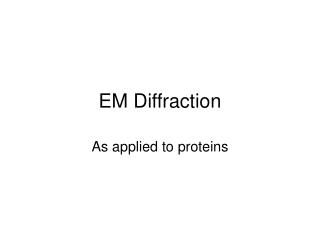DownloadDownload PresentationEM Diffraction

# EM Diffraction

Télécharger la présentation## EM Diffraction

- - - - - - - - - - - - - - - - - - - - - - - - - - - E N D - - - - - - - - - - - - - - - - - - - - - - - - - - -
##### Presentation Transcript

1. EM Diffraction As applied to proteins

2. Diffraction from a grating Molecular Expressions

3. Bragg’s Law • N = 2d∙sin(Ө)/λ • Derivation: http://www.eserc.stonybrook.edu/ProjectJava/Bragg/ • Applies to electron diffraction from crystals • Therefore, the diffraction of electrons from a crystal is quantized

4. Scattering factors • What within the planes of the crystal is scattering electrons? The electrons of the atoms of the molecules. • If we can map the electron density distribution of the scattering material, we can determine crystal structure

5. Fourier Transform We can model any function by adding together waves that are integral multiples in frequency F(x) = C0 + ∑Cncos(2πx/(λ/n) +αn).

6. Scattering of waves by an object gives rise to a Fourier transform • http://micro.magnet.fsu.edu/primer/java/interference/doubleslit/ • The Fourier transform of a single spacing is a single cosine wave (This is how diffraction gratings work) • Note that small spacings in real space give rise to large spacings in reciprocal space • This is the origin of the Rayleigh limit – the cone of scattering that can be collected by a microscope is finite.

7. Inverse Fourier Transform • A Fourier transform of a Fourier transform generates the negative of the original function • We can therefore multiply this by -1 to give an inverse Fourier transform Optically, this is accomplished by a lens • However, all we can measure in the rear focal plane of a microscope is the amplitudes of scattered beams, not their phases • This is the origin of the phase problem in diffraction

8. Electron diffraction crystal structure • Electron diffraction is advantageous because the diffracted electrons average over many repeats of a structure • We can measure diffracted electron intensities • How can we get their phases, so that we can use an inverse Fourier transform to retrieve the structure?

9. Electron Diffraction from BR

10. Electron Crystallography • One way: Do electron diffraction to measure amplitude, and low-dose transmission electron microscopy to get a direct image • Use the direct image to calculate phases • This is extremely useful for proteins that for microcrystals, or two dimensional crystals

11. Flow Chart (Henderson et al, 1990)

12. Examples • 2-D crystals • Purple membrane protein (bacteriorhodopsin) from Halobacter halobium. • Light harvesting complex • http://blanco.biomol.uci.edu/Membrane_Proteins_xtal.html • Microcrystals • Prion protein • Catalase

13. Catalase crystals Brink Lab, Baylor

14. Purple membrane protein (1990)

15. Techniques (Fujiyoshi) • Membranes prepared from Halobacter halobium • Crystals suspended in 3% trehalose • Applied to carbon-coated melybdenum grids • Plunged into liquid ethane to give vitreous ice • Transferred to cryostage of EM (FEG)

16. Projection density map of BR

17. How to access the third dimension?Tilt Series Note that because this is a 2-Dimensional crystal, you are sampling the continuous Fourier transform in the third dimension.

18. Section of 3 Å Electron Density map of BR

19. LHC-II

20. Aquaporin

21. Problems • Stage needs to be chilled with liquid helium (radiation damage) • Tilt series limited to 60° - limits Z resolution • Field emission gun necessary